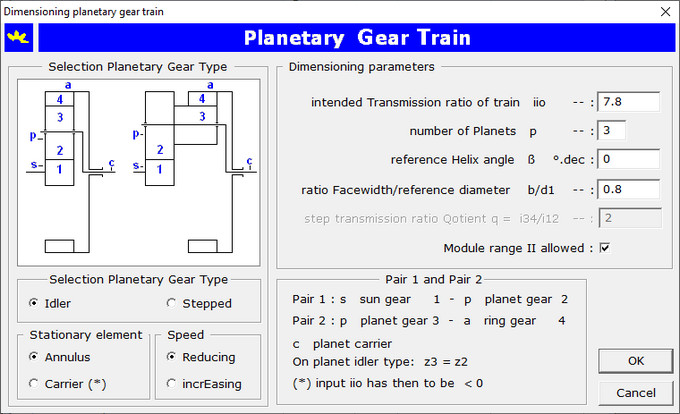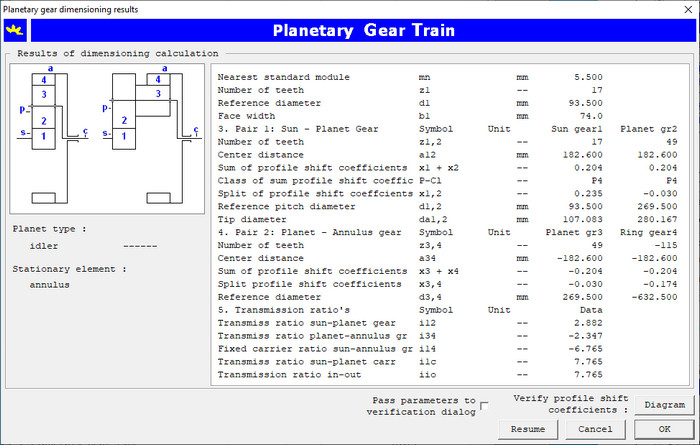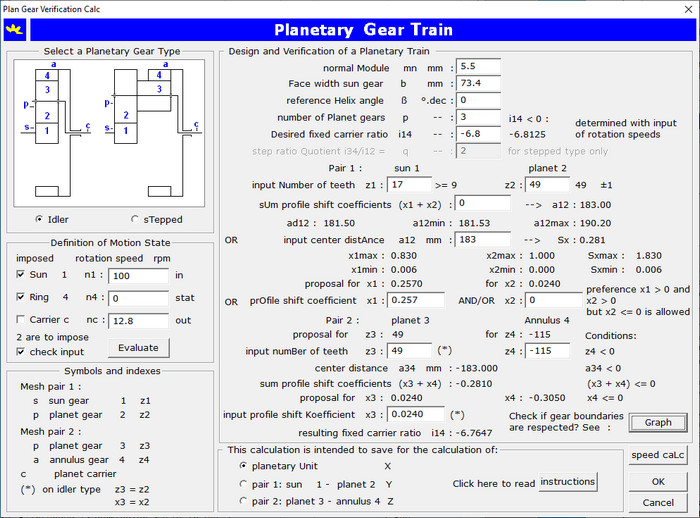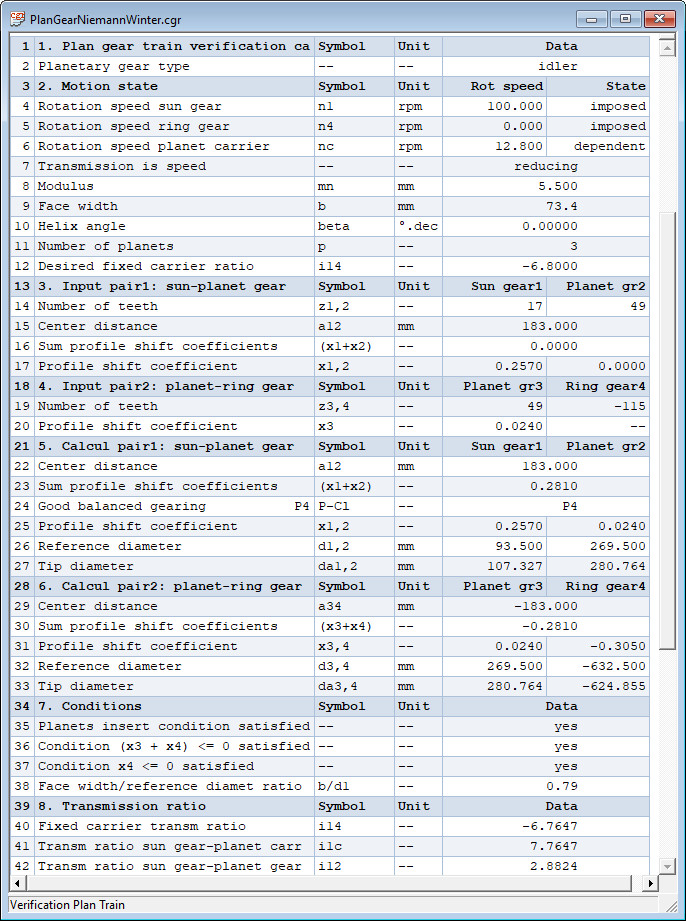## Torque Rating of a Gear Pair

For a given gear geometry, the application determines the torque rating by an iterative calculation process.

## Dimensioning of a Gear Pair

The dimensioning of a gear pair is supported. Input is requested of: transmission ratio, load and the gear materials that will be used. A preference for a slender or compact version can be set. The number of teeth and the gear module are caclulated. The two nearest standard modules are determined in combination with three different number of teeth. This lead to six combinations worth while for further examination.

## Dimensioning a Planetary Gear Train

### Dimensioning for given transmission ratio and load

Selection of materials for sun and planet gear. The material for the annulus is supposed to have characteristics comparable to the material for the planet gear. Input of load and basic planetary parameters as as you can find in following dialog box:The application calculates a solution that match with the specific conditions for a planetary gear train as shown in the following dialog box:### Verification Planetary Gear Train for given module

The calculation is based on the chosen module and on the desired fixed carrier transmission ratio. The user chooses the number of teeth for the sun gear and the application calculates a proposal for the other geometric parameters. The input is to do with following dialog box:The user has to define the motion state of the planetary train: indication for which shafts the rotation speed is to be imposed, followed by their rotation speeds, input of the desired dependent rotation speed for the third shaft. With this input the desired fixed carrier transmission ratio can be calculated as suggestion for the next input of geometric parameters.

Input of geometry: the normal module mn, face width b, reference helix angle β, number of planet gears p, desired fixed carrier transmission ratio i14 and number of teeth of the sun gear z1. With a push on the tab key after each subsequent input, the application will suggest the number of teeth for planet and annulus gears, the center distance a12 for gear pair 1. Start with input of a rounded center distance a12 for pair 1 and calculate the profile shift coefficients for the sun gear, planet gears and ring gear. Try iteratively different values for the center distance until both conditions (x3 + x4) <= 0 and x4 <= 0 are reasonable satisfied.

On clicking the OK button, the calculation results are displayed in a window as follows:Click here on Calculation Report Planetary Gear Train to open an example of a calculation report of a planetary gear train in a target blank page.

End of CylGear web pages. You have now a good idea about the look and the feel of the application. If you are interested go immediately to the Contact Page and ask for a quotation or further information and convince yourself that the application is an excellent tool for calculation of your cylindrical gears on a professional manner.